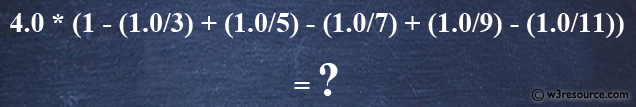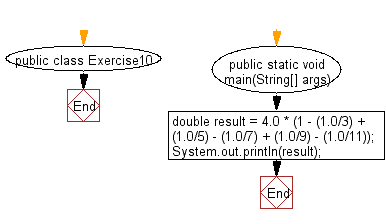﻿ Java exercises: Compute a specified formula - w3resource# Java Exercises: Compute a specified formula

## Java Basic: Exercise-10 with Solution

Write a Java program to compute a specified formula.

Specified Expression :
4.0 * (1 - (1.0/3) + (1.0/5) - (1.0/7) + (1.0/9) - (1.0/11))

Pictorial Presentation:Sample Solution:

Java Code:

``````public class Exercise10 {
public static void main(String[] args) {
double result = 4.0 * (1 - (1.0/3) + (1.0/5) - (1.0/7) + (1.0/9) - (1.0/11));
System.out.println(result); //
}
}
```
```

Sample Output:

```2.9760461760461765
```

Flowchart:Java Code Editor:

What is the difficulty level of this exercise?

Test your Programming skills with w3resource's quiz.

﻿

## Java: Tips of the Day

getEnumMap

Converts to enum to Map where key is the name and value is Enum itself.

```public static <E extends Enum<E>> Map<String, E> getEnumMap(final Class<E> enumClass) {
return Arrays.stream(enumClass.getEnumConstants())
.collect(Collectors.toMap(Enum::name, Function.identity()));
}
```

Ref: https://bit.ly/3xXcFZt